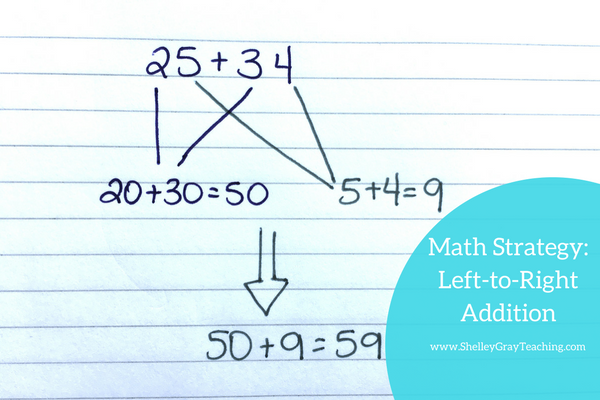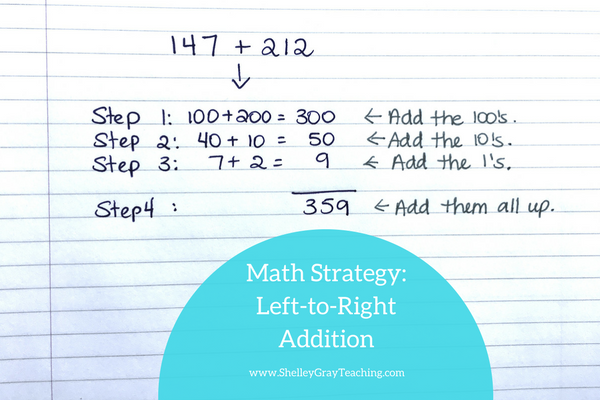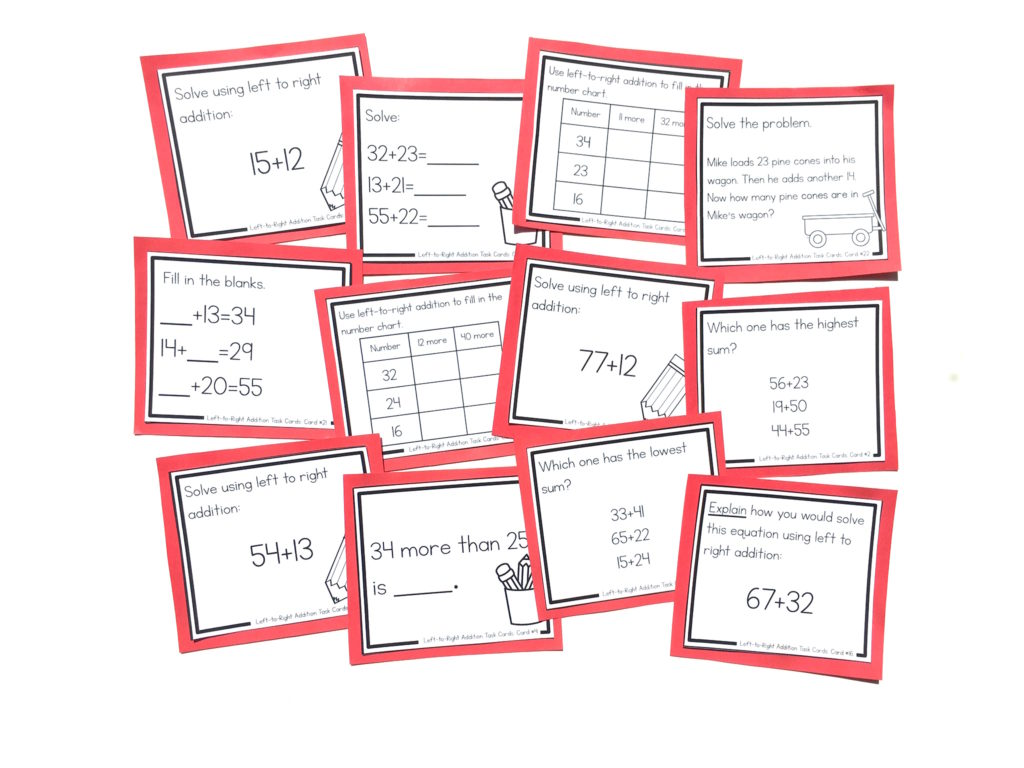Left-to-right addition (also known as front-end addition or the partial sums method) is one of the most powerful mental math strategies for teaching addition of 2 or 3-digit numbers. However, many people are confused by why it is important and why it can be more effective than traditional vertical addition.

WHY IS IT SO EFFECTIVE?

The best part about left to right addition is that this strategy promotes real understanding.

When you solve an equation using the standard algorithm (probably the way that you learned to add multi-digit numbers), you use a series of steps. This includes adding the ones first, carrying if needed, then adding the tens, carrying if needed, etc. These steps are committed to your memory, and for those who have excellent memorization skills, this can be effective.

HOWEVER, the standard algorithm does not encourage understanding of place value and number sense. This is the main reason that today’s math instruction tends to shy away from the traditional algorithm. We want our students to possess REAL understanding of what they are doing. When students are taught methods that encourage mental math, they are able to think more flexibly not only about this isolated concept, but about other math concepts as well.

EXAMPLES

Let’s take a look at some examples of left-to-right addition in action.

In this example we are adding 25+34. First, we add the tens: 20+30, to make 50. Then we add the ones: 5+4, to make 9. Lastly, we add 50+9 to make 59. Although this may look confusing to have it written out as it is, this process happens very quickly once a student understands the process – typically an equation like this will be solved in a couple of seconds at the most.Left to right addition is also effective for adding 3-digit plus 3-digit numbers. In this example, we can see that we add the hundreds first, then the tens, and then the ones. Lastly, we add all of those sums together.When students perform addition this way, they develop a good understanding of place value and what it really means. For example, in the equation shown above, students see the “1” in 147 as a 100, and not just a 1. The “4” in 147 is understood as a 40, and not just a 4. This is essential knowledge if we want our students to become efficient mathematicians.

NEXT STEPS:

• If you would like full support for teaching addition strategies in your classroom, check out The Addition Station HEREYou’ll find the left to right addition strategy in the Addition Stations for the upper grades. These Math Stations are self-paced, student-centered stations for the basic math strategies. Students move through the levels at their own pace, ensuring that they are always challenged, and working to their full potential.• Check out a “Front End Addition Mental Math Strategy Unit” HERE.
•  Find task cards to reinforce the left-to-right addition strategy in isolation here:•Do you have similar posts for subtraction strategies?

•Hi Cindy! I don’t have Subtraction Strategy posts at this time, but they are on my to-do list for the next couple of months! 🙂

Have a great day,

Shelley

•